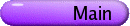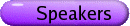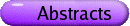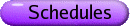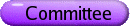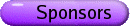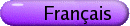Nonlinear Analysis Org: Martial Agueh (Victoria), Ivar Ekeland (PIMS) and Robert McCann (Toronto)[PDF] RUSTUM CHOKSI, Simon Fraser University The Periodic Isoperimetric and Cahn-Hilliard Problems and Nonlocal Perturbations [PDF] I will discuss two classical problems in analysis and geometry posed on n-dimensional flat torus. I will focus on some interesting questions which remain open. I will then discuss a nonlocal isoperimetric problem with an emphasis on the calculation and application of its first and second variations. This talk is based on joint work with Peter Sternberg (Indiana University). DANIELA DE SILVA, MSRI, 17 Gauss Way, Berkeley, CA 94720, USA A singular energy minimizing free boundary [PDF] In this talk, we consider the problem of minimizing the energy functional ò(|Ñu|2 + c{u > 0}). We will exhibit the first example of a singular energy minimizer, which occurs in dimension n=7. This is the analogue of the 8-dimensional Simons cone in the theory of minimal surfaces. This is joint work with D. Jerison. IVAR EKELAND, University of British Columbia A new type of equation arising from noncommitment in economics [PDF] In control theory one traditionally uses an exponential discount factor to arbitrage future gains agains current losses. It is well known that the optimal control problem then is solved by a Hamilton-Jacobi-Bellman equation for the value function. In joint work with Ali Lazrak, we point out that this approach completely breaks down when the discount is not exponential, and the decision-maker cannot commit. Instead of looking for optimal controls, one must then look for equilibrium strategies, and the HJB equation is then replaced by a remarkable integro-differential equation. PIERPAOLO ESPOSITO, UBC, PIMS, 1933 West Mall, Vancouver, BC V6T 1Z2 On a semilinear PDE with a singular nonlinearity [PDF] I will discuss some recent progress on the semilinear elliptic problem Du = [(lf(x))/((1+u)2)] on a smooth bounded domain W of RN with an homogeneous Dirichlet boundary condition. This equation models a simple electrostatic Micro-Electromechanical System (MEMS) device and has been studied recently by Pelesco, and by Guo-Pan-Ward. Guo and Ghoussoub show-among other things-that the branch of minimal solutions ul is compact up to a certain critical value l*, provided 1 £ N £ 7. In this talk, I will describe an analogous result for the second branch (of "mountain pass" solutions), which holds in the same low dimensions. Our techniques rely on a careful blow-up analysis for solutions satisfying certain spectral properties. Joint work with N. Ghoussoub and Y. Guo. RICHARD FROESE, University of British Columbia AC spectrum for the Anderson model on a tree: a geometric proof [PDF] We give a new proof of Klein's result on the existence of absolutely continuous spectrum for a discrete random Schrödinger operator on a tree with small disorder. Our proof relies on a new geometric way of controlling the Green's function, based on the contraction properties of a transformation in hyperbolic space. This is joint work with David Hasler and Wolfgang Spitzer. NASSIF GHOUSSOUB, University of British Columbia Concentration estimates for Emden-Fowler equations with boundary singularities and critical growth [PDF] We establish existence and multiplicity of solutions for the Dirichlet problem åi ¶ii u + [(|u|2*-2u)/(|x|s)] = 0 on smooth bounded domains W of Rn (n ³ 3) involving the critical Hardy-Sobolev exponent 2* = [(2(n-s))/(n-2)] where 0 < s < 2, and in the case where zero (the point of singularity) is on the boundary ¶W. Just as in the Yamabe-type non-singular framework (i.e., when s=0), there is no nontrivial solution under global convexity assumption (e.g., when W is star-shaped around 0). However, in contrast to the non-satisfactory situation of the non-singular case, we show the existence of an infinite number of solutions under an assumption of local strict concavity of ¶W at 0 in at least one direction. More precisely, we need the principal curvatures of ¶W at 0 to be nonpositive but not all vanishing. We also show that the best constant in the Hardy-Sobolev inequality is attained as long as the mean curvature of ¶W at 0 is negative. The key ingredients in our proof are refined concentration estimates which yield compactness for certain Palais-Smale sequences which do not hold in the non-singular case. This is joint work with Frederic Robert. STEPHEN GUSTAFSON, UBC Dept. of Mathematics, 1984 Mathematics Rd., Vancouver, BC V6T 1Z2 Schroedinger maps near harmonic maps [PDF] The Schroedinger map equation is a basic model in ferromagnetism, as well as a geometric (and hence non-linear) version of the linear Schroedinger equation. An important open question is whether finite energy solutions are globally smooth, or blow up in finite time. We describe some results for equivariant Schroedinger maps from 2+1-dimensional space-time into the 2-sphere, with energy close to the energy of harmonic maps. This is joint work with Kyungkeun Kang and Tai-Peng Tsai. DAVID HARTENSTINE, Western Washington University Regularity of Weak Solutions of the Monge-Ampère Equation [PDF] Regularity properties of Aleksandrov solutions to the Dirichlet problem for the Monge-Ampère equation detD2 u = m where m is a Borel measure on a convex domain in Rn will be discussed. The measure m satisfies a condition, introduced by Jerison, that is weaker than the doubling condition. Some of the results of Caffarelli's regularity theory for the Monge-Ampère equation, more specifically strict convexity and interior C1,a regularity, are extended to the solutions of these problems. This is joint work with Cristian Gutierrez. RICHARD KENYON, UBC, Vancouver Dimers and the complex Burgers equation [PDF] We study a simple model of crystalline surfaces in R3. Microscopically, these are random discrete surfaces, arising in the so-called dimer model, or domino tiling model. The law of large numbers implies that at large scales the surfaces take on definite shapes, which are smooth surfaces satisfying a certain PDE, related to the complex Burgers equation. We show how this equation can be solved via complex analytic functions, and investigate the behavior of solutions, in particular the formation of facets. This is the first model of facet formation which can be analytically solved. This is joint work with Andrei Okounkov. RUEDIGER LANDES, University of Oklahoma, Norman, OK 73071 Wave front solutions in the theory of boiling liquids [PDF] In an new attempt to model the phase transition from nucleate to transient boiling, Professor Marquardt from Aachen proposed to consider the the heat flow in the heating vessel rather than in the boiling liquid. In this model the heat flow in the wall of the vessel, subject to heat equation, is combined with a nonlinear Neumann boundary condition at the surface of the wall toward the boiling liquid. The nonlinearity is determined by the change of the heat conduction coefficient in the phase transition. For the one-dimensional heat equation with a nonlinear inhomogeneous term, the existence of wavefront solutions is well known and widely used to model phase transitions. Work of Aronson and Weinberger also dealt with the more-dimensional situation and showed that there are sub-solutions which behave like wavefronts. Consequently the actual solutions must have a sudden change of state, also. However, this model with the nonlinearity in the equation rather then the boundary condition can be justified in our case for (infinitely) thin surfaces only. Here we present an approach which provides a wavefront type sub-solution for the nonlinear Neumann problem and hence establish a first mathematical confirmation of Marquardt's model. Further research will concentrate on the discussion of initial configurations (dry spots) which generate a wavefront type solutions and those which do not. In addition we intend to address the question how the maximum and minimum speed of the traveling wave is determined by the initial configuration and the other parameters of the data. ABBAS MOAMENI, University of British Columbia Self-dual variational principles for periodic solutions of Hamiltonian and other dynamical systems [PDF] Self-dual variational principles are introduced in order to construct solutions for Hamiltonian and other dynamical systems which satisfy a variety of linear and non-linear boundary conditions including many of the standard ones. These principles lead to new variational proofs of the existence of parabolic flows with prescribed initial conditions, as well as periodic, anti-periodic and skew-periodic orbits of Hamiltonian systems. This is a joint work with N. Ghoussoub. TRUYEN NGUYEN, Mathematical Sciences Research Institute, 17 Gauss Way, Berkeley, CA 94720-5070 On Monge-Ampère Type Equations Arising In Optimal Transportation Problems [PDF] In this talk, we will discuss Monge-Ampère type equations arising in optimal transportation problems. We prove the comparison principle, maximum principle and also a quantitative estimate of Aleksandrov type for c-convex functions. These results are in turn used to prove the solvability and uniqueness of weak solutions for the Dirichlet problems. ADAM OBERMAN, SFU Numerical methods for Mass Transportation [PDF] Numerical results for the mass tranportation problem will be presented. The transportation problem with cost function which depend on the difference x-y will be considered. We approximate measures by atoms, and project to a finite dimensional linear programming problem, which can then be solved by standard methods. Numerical results for linear, quadratic, and square root of distance costs will be presented. We will also present a convergent finite difference method for solving the Dirichlet problem for the Monge-Ampère equation. GUNTHER UHLMANN, University of Washington The Calderon problem with partial data [PDF] We will consider in this talk the inverse problem of determining the electrical conductivity inside a medium by making voltage and current measurements on subsets of the boundary. We will state and outline the proofs of some recent results on this problem. MICHAEL WARD, Dept. of Mathematics, University of British Columbia, Vancouver, BC Eigenvalue Optimization, Spikes, and the Neumann Green's Function [PDF] An optimization problem for the fundamental eigenvalue of the Laplacian in a planar simply-connected domain that contains N small identically-shaped holes, each of a small radius e << 1, is considered. The boundary condition on the domain is assumed to be of Neumann type, and a Dirichlet condition is imposed on the boundary of each of the holes. The reciprocal of this eigenvalue is proportional to the expected lifetime for Brownian motion in a domain with a reflecting boundary that contains N small traps. For small hole radii e, we derive an asymptotic expansion for this eigenvalue in terms of the hole locations and the Neumann Green's function for the Laplacian. For the unit disk, ring-type configurations of holes are constructed to optimize the eigenvalue with respect to the hole locations. For a one-hole configuration, the uniqueness of the optimizing hole location in symmetric and asymmetric dumbbell-shaped domains is investigated. This eigenvalue optimization problem is shown to be closely related to the problem of determining certain vortex configurations in the Ginzburg-Landau theory of superconuctivity and to the problem of determining equilibrium locations of particle-like solutions, called spikes, to certain singularly perturbed nonlinear reaction-diffusion systems. Some results for the equilibria, stability, and bifurcation behavior, of these spike solutions are given.